# Monte Carlo simulation for estimating slope failure risk¶

This notebook is an element of the free risk-engineering.org courseware. It can be distributed under the terms of the Creative Commons Attribution-ShareAlike licence.

Author: Eric Marsden eric.marsden@risk-engineering.org

This notebook contains an introduction to use of Python and the NumPy library for Monte Carlo simulation applied to the estimation of the stability of natural slopes (basic predictions of landslide risk). See the associated course materials for background on stochastic simulation methods and to download this content as a Jupyter/Python notebook.

Acknowledgement. This notebook is an adaptation of an exercise from the MIT course “Probability and statistics in engineering” taught by Prof. Daniele Veneziano (application example 17).

## Predicting landslide risk¶

The possible instability of natural slopes is a major geological hazard in many hilly or mountainous regions of the world. It can lead to landslides that sweep away houses and vehicles, and at a smaller scale the collapse of embankments along railway lines can lead to derailments, or to dike failures and flooding. The degree of stability of a slope may be expressed through the slope stability factor $F$, which is the ratio between a soil resistance measure and the associated applied load. Hence values of $F$ larger than 1 are associated with stability and values smaller than 1 indicate unstable conditions. For natural slopes with a shallow soil layer, the slope stability factor is given by (Skempton and DeLory, 1957):

$F = \frac{c}{\gamma z \sin(\alpha) \cos(\alpha)} + \frac{\tan(\phi)}{\tan(\alpha)} - m \frac{\gamma_w \tan(\phi)}{\gamma \tan(\alpha)}$

where:

• $c$ is the cohesion of the soil
• $\phi$ is the angle of internal friction of the soil
• $\gamma$ is the unit weight of the soil
• $\gamma_w$ is the unit weight of water
• $\alpha$ is the inclination of the slope to the horizontal, larger than 0
• $z$ is the depth below the ground surface
• $z_w$ is the depth of the water table below the ground surface
• $m$ is the degree of saturation of the soil, where $m = \frac{z_w - z}{z}$

This is called the infinite slope stability model.

The failure criterion is $F < 1$. Areas with $1 < F < 1.25$ are said to be moderately susceptible to landslide risk, with $1.25 < F < 1.5$ slightly susceptible and not susceptible (or stable) for $F > 1.5$.

With the following typical values for the parameters, we have $F = 1.15$, meaning the slope is moderately susceptible.

In :
import math
from typing import NamedTuple

# here we use some modern Python features that help detect typos
class Params(NamedTuple):
z: float
z_w: float
m: float
c: float
phi: float
alpha: float
gamma: float
gamma_w: float

def default_params() -> Params:
return Params(z=5,
z_w = 2.5,
m = 0.5,
c = 25,
gamma = 20,
gamma_w = 9.81)

def slope_stability(p: Params):
c1 = p.c / (p.gamma * p.z * math.sin(p.alpha) * math.cos(p.alpha))
c2 = math.tan(p.phi) / math.tan(p.alpha)
c3 = p.m * p.gamma_w * math.tan(p.phi) / (p.gamma * math.tan(p.alpha))
return c1 + c2 - c3

slope_stability(default_params())

Out:
1.1544116861109888

To check our intuitive understanding of landslide risk, let’s check that risk increases ($F$ goes down) as the slope increases between 0 and 90° (maintaining all other inputs at their central values), and also that the risk increases as the amount of water in the soil increases.

In :
import numpy
import matplotlib.pyplot as plt
plt.style.use("bmh")

def slope_stability_alpha(alpha: float) -> float:
params = default_params()

support = numpy.linspace(1, 45, 100)
outputs = numpy.array([slope_stability_alpha(a) for a in support])

In :
plt.plot(support, outputs)
plt.axhline(y=1, color="r")
plt.xlabel("Slope (α)")
plt.ylabel("Slope stability factor (F)");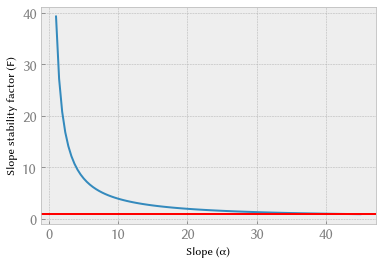In :
def slope_stability_m(m: float) -> float:
params = default_params()
return slope_stability(params._replace(m=m))

support = numpy.linspace(0.05, 0.95, 100)
outputs = numpy.array([slope_stability_m(m) for m in support])

In :
plt.plot(support, outputs)
plt.axhline(y=1, color="r")
plt.xlabel("Soil water saturation (m)")
plt.ylabel("Slope stability factor (F)");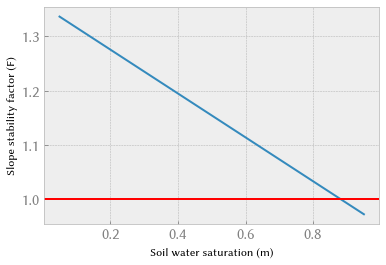## Uncertainty analysis¶

The main uncertain parameters are $c$ and $\phi$, with coefficients of variation respectively of 0.2 and 0.25. Experience shows that they can be assumed to be normally distributed. To run a stochastic simulation, we define a function that generates one random set of inputs to the stability calculation.

In :
import scipy.stats

# CV = sigma / mu
def randomize_params(params: Params):
return params._replace(c=scipy.stats.norm(25, 0.2*25).rvs(),

randomize_params(default_params())

Out:
Params(z=5, z_w=2.5, m=0.5, c=23.276701155312505, phi=0.3699693853201186, alpha=0.6108652381980153, gamma=20, gamma_w=9.81)

We can now run a Monte Carlo simulation to estimate the distribution of the safety factor $F$.

In :
import numpy

N = 10_000
sim = numpy.zeros(N)
failed = 0
for i in range(N):
sim[i] = slope_stability(randomize_params(default_params()))
if sim[i] < 1:
failed += 1
print("Estimated failure probability: {:.3f}".format(failed / float(N)))

Estimated failure probability: 0.222


Recall that simulations with a slope stability factor less than 1 (to the left of the red line in the histogram below) represent failures (landslides).

In :
plt.hist(sim, bins=40, density=True, alpha=0.5)
plt.axvline(x=1, color="r")
plt.xlabel("Slope stability factor");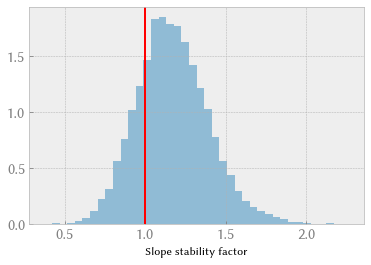We can estimate the failure probability as a function of the slope.

In :
def failure_probability_alpha(alpha: float) -> float:
N = 1000
failed = 0
for i in range(N):
params = randomize_params(default_params())
if slope_stability(params) < 1:
failed += 1
return failed / float(N)

support = numpy.linspace(1, 55, 20)
outputs = numpy.array([failure_probability_alpha(a) for a in support])

In :
plt.plot(support, outputs)
plt.xlabel("Slope (alpha)")
plt.ylabel("Failure probability");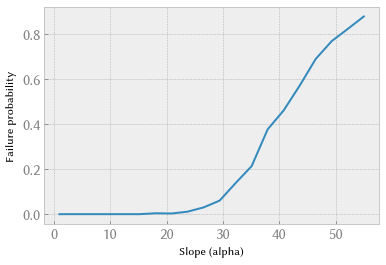Similarly, we can plot failure probability as a function of the soil’s water saturation.

In :
def failure_probability_m(m: float) -> float:
N = 1000
failed = 0
for i in range(N):
params = randomize_params(default_params())
if slope_stability(params._replace(m=m)) < 1:
failed += 1
return failed / float(N)

support = numpy.linspace(0.05, 0.95, 20)
outputs = numpy.array([failure_probability_m(m) for m in support])

In :
plt.plot(support, outputs)
plt.xlabel("Soil water saturation (m)")
plt.ylabel("Failure probability");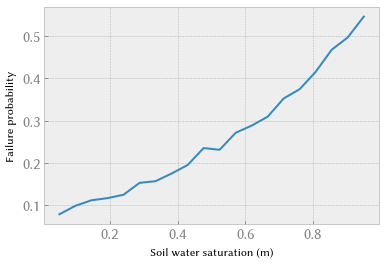In [ ]: